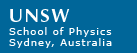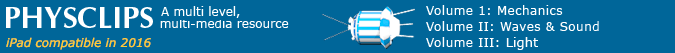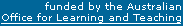# Newton's laws: more background

Newton's laws are introduced in a multimedia tutorial: Chapter 5 of Physclips. This page gives some deeper and broader background. In particular, it addresses the philosophical basis and limitations to Newtons laws.

### Galileo's argument

The argument upon which Galileo's mechanics is based is represented here. A ball in a bowl rolls down one side and up the other, where it stops when it has reached (almost) the same height. The 'almost' is required because of air resistance and rolling resistance: in the absence of these small complications we expect that it would reach the same height.

If we make the bowl asymmetric, as in the animation, it has to roll further to reach the original height, but it does so, in the absence of the complications acknowledged. So, if we make the path completely flat, and again neglecting those complications, the ball would roll for ever.

To Aristotle, the 'natural' state of matter was to be stationary: v = 0. To Galileo, the 'natural' state of matter was moving in a straight line at constant speed: i.ev = constant.

### What happens next? How does the past influence the future?

How does past motion influence the future? Does the earth go around the sun because it has been doing so in the past? If we remove all external effects, what properties of the past and current motion are preserved into the future?

In this experiment, the ball travels in a curved path, because the wall exerts a horizontal force that acts roughly towards the centre of the spiral. When the ball leaves the spiral and the wall is no longer there to exert a horizontal force, what will be the new path of the ball? Straight line or curve? Run the video to find out.

### The units of force: how big is a newton?

Mel Fyfe, the strong woman from Circus Oz supports the weight of four other people, as well as her own. The total weight is probably about 4 kilonewtons, which is the force her feet (together) exert on the floor and that of course the floor exerts on her.

A kilonewton is a reasonably large force for a human to exert, but by no means the maximum.

The weight of the feather resting on my hand is about a millinewton, and I could feel it. Much smaller forces are hard to feel. Still, from mN to kN is a range of a million, and we exceed this range comfortably.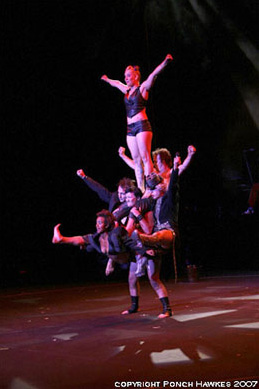Mel Fyfe, Photo courtesy Circus Oz.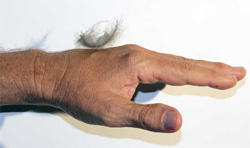### The units of force: some more examples

My weight (near the earth) is 700 newtons. A small peach or a small apple has a weight of about 1 newton. You can make some smaller weights with scissors and typical printer paper, as shown here.### Strings, springs and chains

The phrase 'light, inextensible string' occurs often in problems that illustrate Newton's laws. What does it mean?

 'Inextensible' means that its length doesn't change. A consequence of that is that, if it is straight, the left hand end and the right hand end have the same acceleration. In the video at left, my hand and the trolley therefore have the same acceleration. In the middle video, a rubber band is used. It is not inextensible, so the two accelerations are not equal. 'Light' means that its mass is negligible compared with the other masses in the problem. Consider the tension in the string at the right hand end, with magnitude Tright and at the left hand end, with magnitude Tleft. Here, Tleft accelerates the trolley (mass M). If the acceleration is a, then Tleft = Ma Tright accelerates both the trolley (mass M) and the string (mass m), so Tright = (M + m)a So, if m << M, then Tright  ≅   Tleft . This is useful because it simplifies analysis slightly in many cases. In the example at right, a chain is used. Here, it would be inappropriate to make the approximation m << M, so, during the acceleration phase, Tright  ≠   Tleft .This work is licensed under a Creative Commons License.